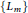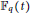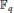Home

# Regulators of an Infinite Family of the Simplest Quartic Function Fields

## Abstract

We explicitly find regulators of an infinite family$\{{{L}_{m}}\}$ of the simplest quartic function fields with a parameter$m$ in a polynomial ring${{\mathbb{F}}_{q}}\left( t \right)$ , where${{\mathbb{F}}_{q}}$ is the finite field of order$q$ with odd characteristic. In fact, this infinite family of the simplest quartic function fields are subfields of maximal real subfields of cyclotomic function fields having the same conductors. We obtain a lower bound on the class numbers of the family$\{{{L}_{m}}\}$ and some result on the divisibility of the divisor class numbers of cyclotomic function fields that contain$\{{{L}_{m}}\}$ as their subfields. Furthermore, we find an explicit criterion for the characterization of splitting types of all the primes of the rational function field${{\mathbb{F}}_{q}}\left( t \right)$ in$\{{{L}_{m}}\}$ .

## References

Hide All
MathJax
MathJax is a JavaScript display engine for mathematics. For more information see http://www.mathjax.org.

# Regulators of an Infinite Family of the Simplest Quartic Function Fields

## Metrics

### Full text viewsFull text views reflects the number of PDF downloads, PDFs sent to Google Drive, Dropbox and Kindle and HTML full text views.

Total number of HTML views: 0
Total number of PDF views: 0 *Loading metrics...

### Abstract viewsAbstract views reflect the number of visits to the article landing page.

Total abstract views: 0 *Loading metrics...

* Views captured on Cambridge Core between <date>. This data will be updated every 24 hours.

Usage data cannot currently be displayed.